Introduction    Graphs    Blog    Theory    About    Contact

## Theory (first draft December 26, 2020)

Disclaimer: none of this has been peer-reviewed.

My credentials allow me to make statements about math, but not medicine.
My goal is to explain the math to you so that you can draw your own
conclusions about the state of the public heath threat.

### Farr's Law, 1840

 From Forbes Magazine: "In what's called 'Farr's Law,' dating back to 1840, all epidemics follow a roughly bell-shaped curve. As the most vulnerable victims are nabbed first, one existing case leads to more than one. But eventually each new infection causes only one other, and then less than another. That's why ultimately all, since before the Plague of Athens (433 BC) are self-limiting. They peak and fall without benefit of the CDC, WHO, vaccines or effective treatments." (Forbes, Oct 23, 2014).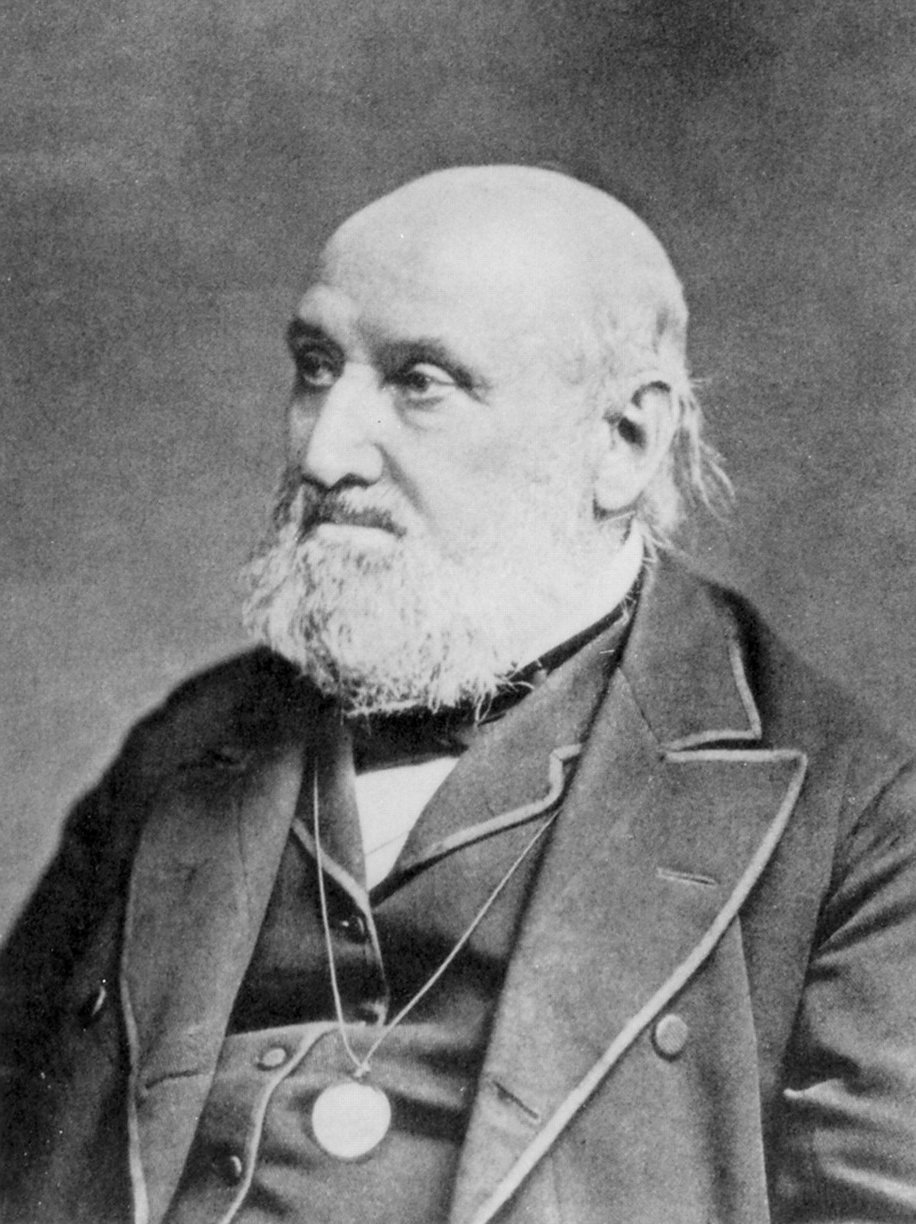Bell Curve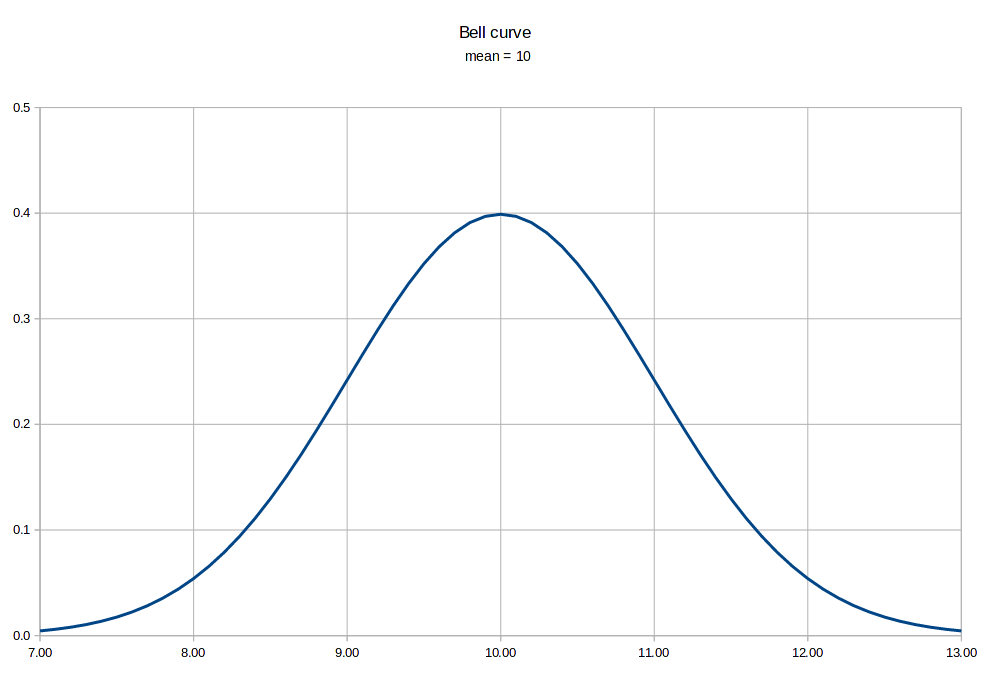Logistics curve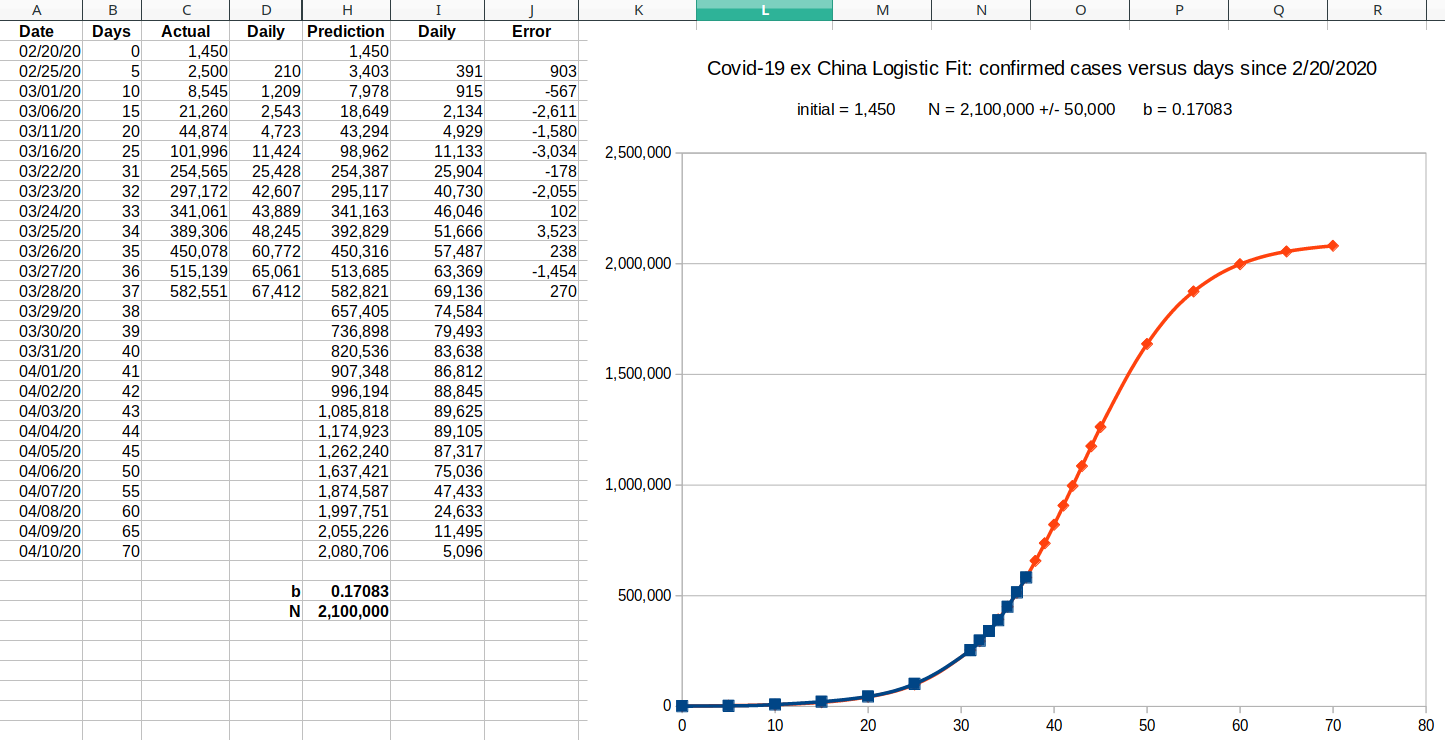The normal distribution, also called the Bell Curve, is is closely related to the logistics curve (or "S" curve), which is often used to model resource-limited growth. Basically the logistics curve is just the summation or integral of the normal distribution. When used for epidemics, the resource that is limited is the number of susceptible people. Once everyone who can get the virus has been infected, there is no way to make a new case.

The second chart shows a logistics fit to the Covid-19 data from the United States on March 28th. There are three variables on a logistics curve, the limit N, the growth rate beta (or sometimes k), and an x value, usually the centerpoint of the curve.

Note that the S-curve becomes linear at exactly half of the limit value N... so in theory if you're watching an epidemic as it progresses, when the growth peaks, you're halfway through.

One important filter step is to use a 7-day average of the daily case and death data, to eliminate the weekly oscillations caused by office closures on weekends. Once you do that, the scattered data points settle into a nice line.

 Daily new cases, United States, May 5, 2020Daily new cases, World ex-China, April 22, 2020In the case of the coronavirus, the linear portion of the curve seemed to stretch out longer than a regular logistics curve. Also the predicted maxmimum number of cases N was only two million, which seemed way too low. As April numbers came in, the regular logistic model just didn't work.

### The skew-normal distribution

My "eureka" moment came on May 16, 2020 when I realized that my earlier logistic models from April didn't fit because the daily changes were not symmetrical. The curve was skewed, which is a standard measure in statistics.

 Negative skew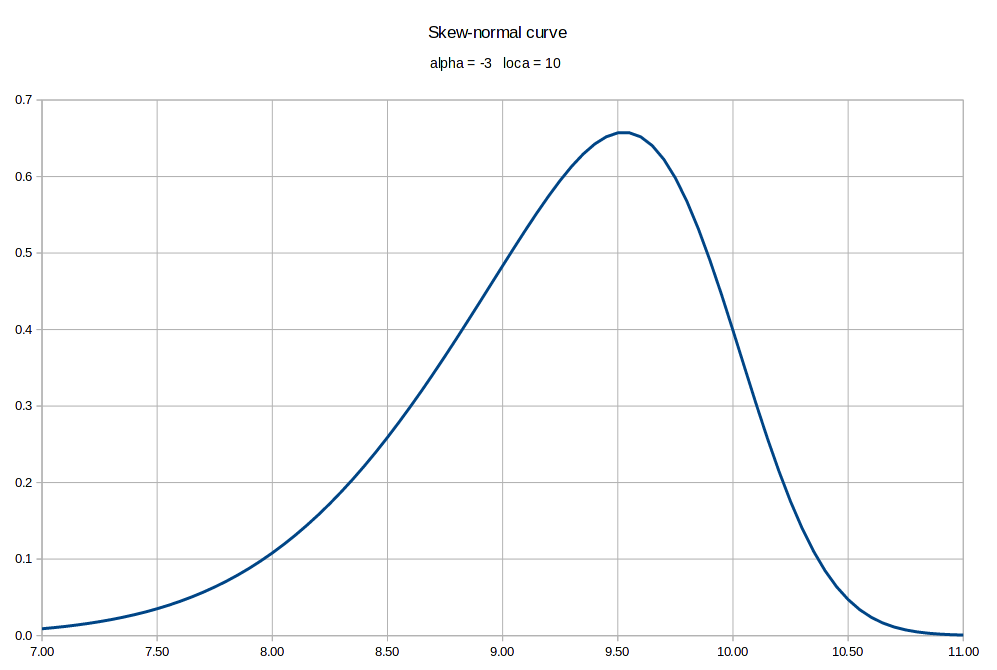Zero skew or Normal curvePositive skewA skew-normal curve is specified by four parameters which change the shape of the standard bell curve:
• alpha: the degree of skewness (α)
• loca: an X offset parameter (ξ)
• scale X: a width scale factor (ω)
• scale Y: a height scale factor
Skewness can be thought of as having the area under the curve greater to one side or the other of the peak. If the larger area is to the right of the peak, the curve is said to have positive skew. If the larger area is to the left of the peak, the curve is said to have negative skew. A symmetric curve has zero skew.

Additionally you could add kurtosis, which some describe as how pointy the curve is, but it really describes how fat the tails of the distribution are. I did not find a need to add a kurtosis parameter to fit the curves from this pandemic.

The Wikipedia article on the skew-normal distribution is very good. I've copied the mathy formulae here:

 standard normal probability density function phi AKA ø function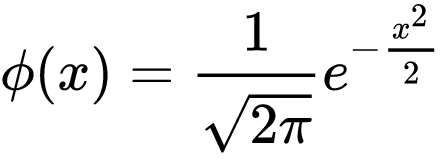cumulative distribution function Φ functionThe skew normal function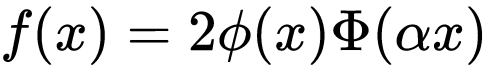The X position and width scaling equation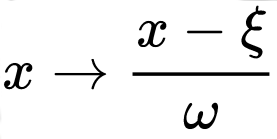Fortunately phi(x) and erf(x) are both available as primitives in spreadsheet programs. The C math library provides erf(x), and phi(x) is easy to write. Here is the skew-normal function written for LibreOffice Calc:

y = scaley * PHI((x-loca)/scalex) * (1 + ERF(alpha * ((x-loca)/scalex)/SQRT(2)))

Getting a decent curve fit for a single wave pulse is easy to do by hand. That's how these curves were done, from May 27th:

 Daily new cases, US, May 27, 2020Daily deaths, US, May 27, 2020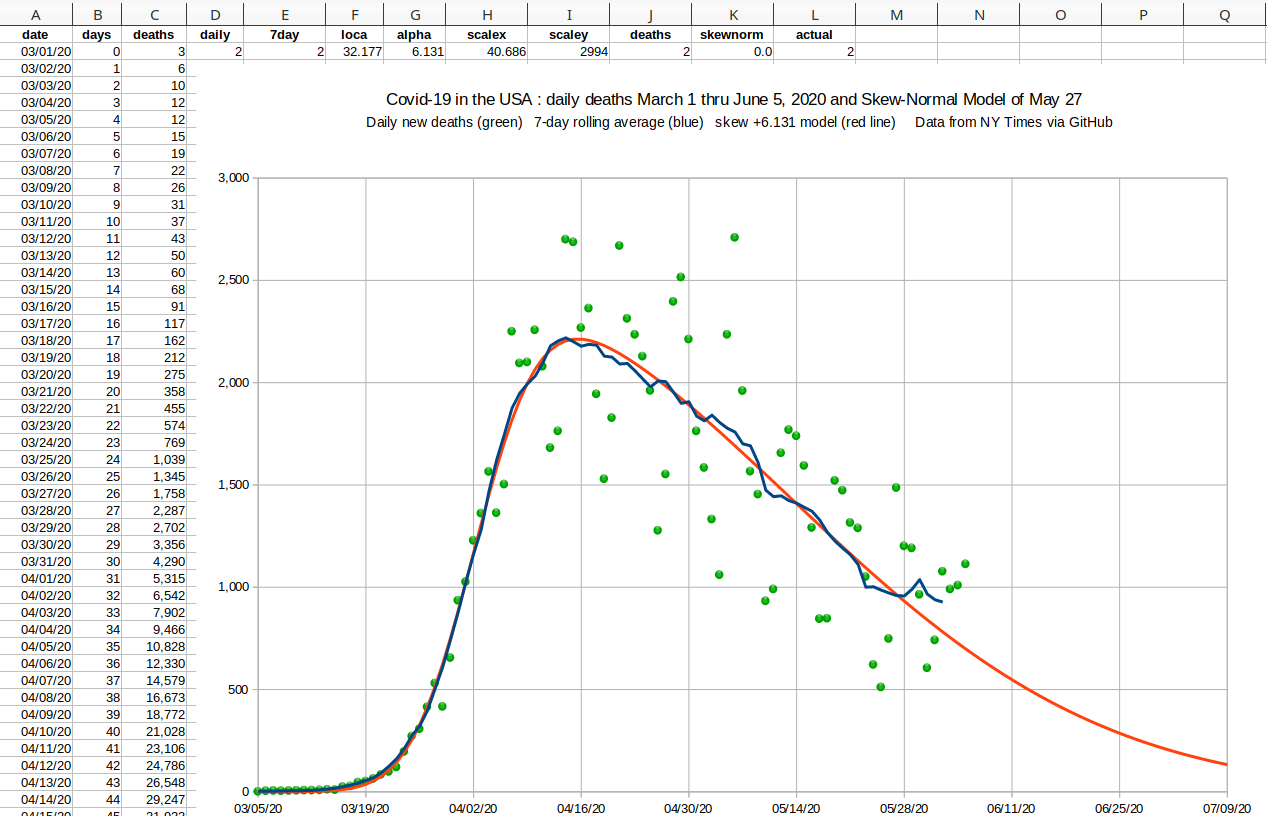### Brute Force

To reduce the amount of time I spent fiddling with the parameters in the spreadsheet, I coded a simple C program to do a uniform search through a small range for each of the four parameters. This is basically just a brute force method.

Then in summer of 2020 both cases and deaths started to rise above the trend modeled by the skew-normal model. By mid-August there was an obvious peak in this "second wave", and I needed a way to model the curves.

The obvious answer is to go from a four-tuple to an eight-tuple, and I modified my brute force optimizer to work in eight dimensions. An eight-level deep nest of for loops is an all time high for my programming career.

 Four dimensional search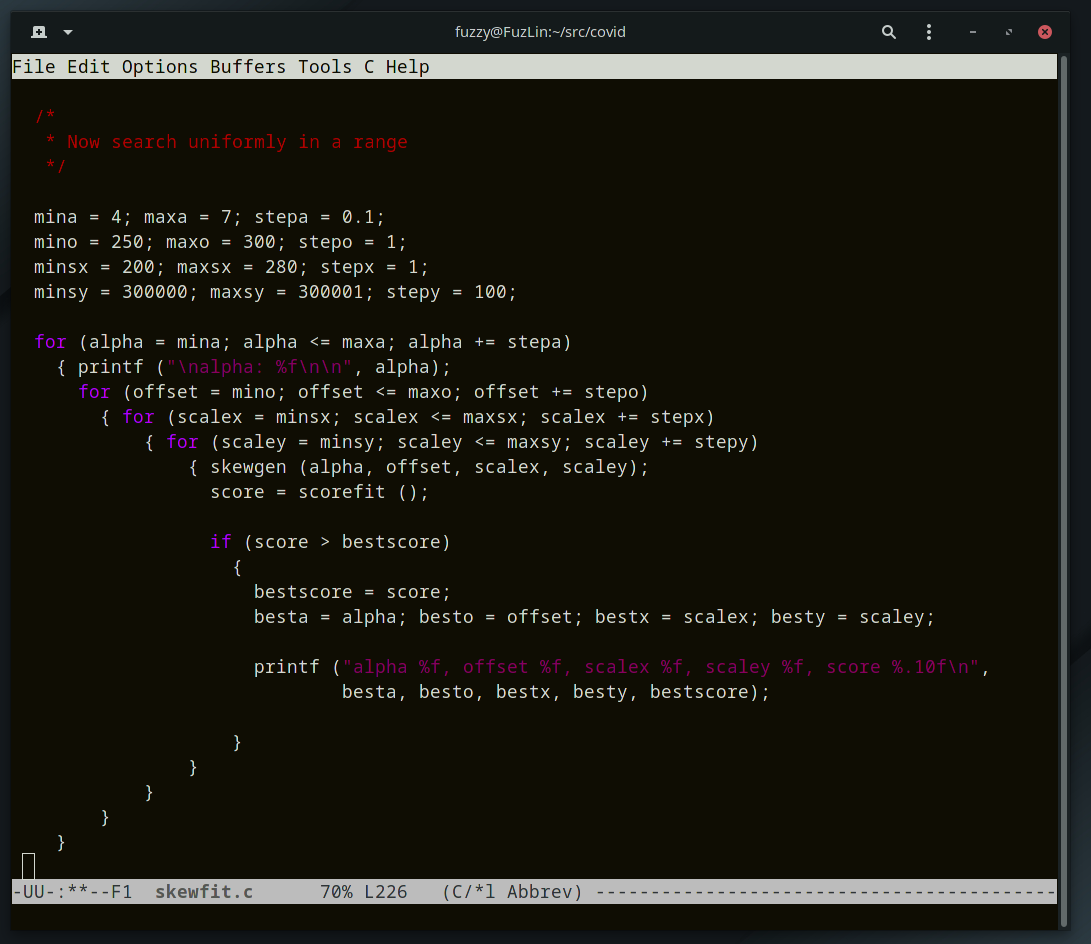Eight dimensional search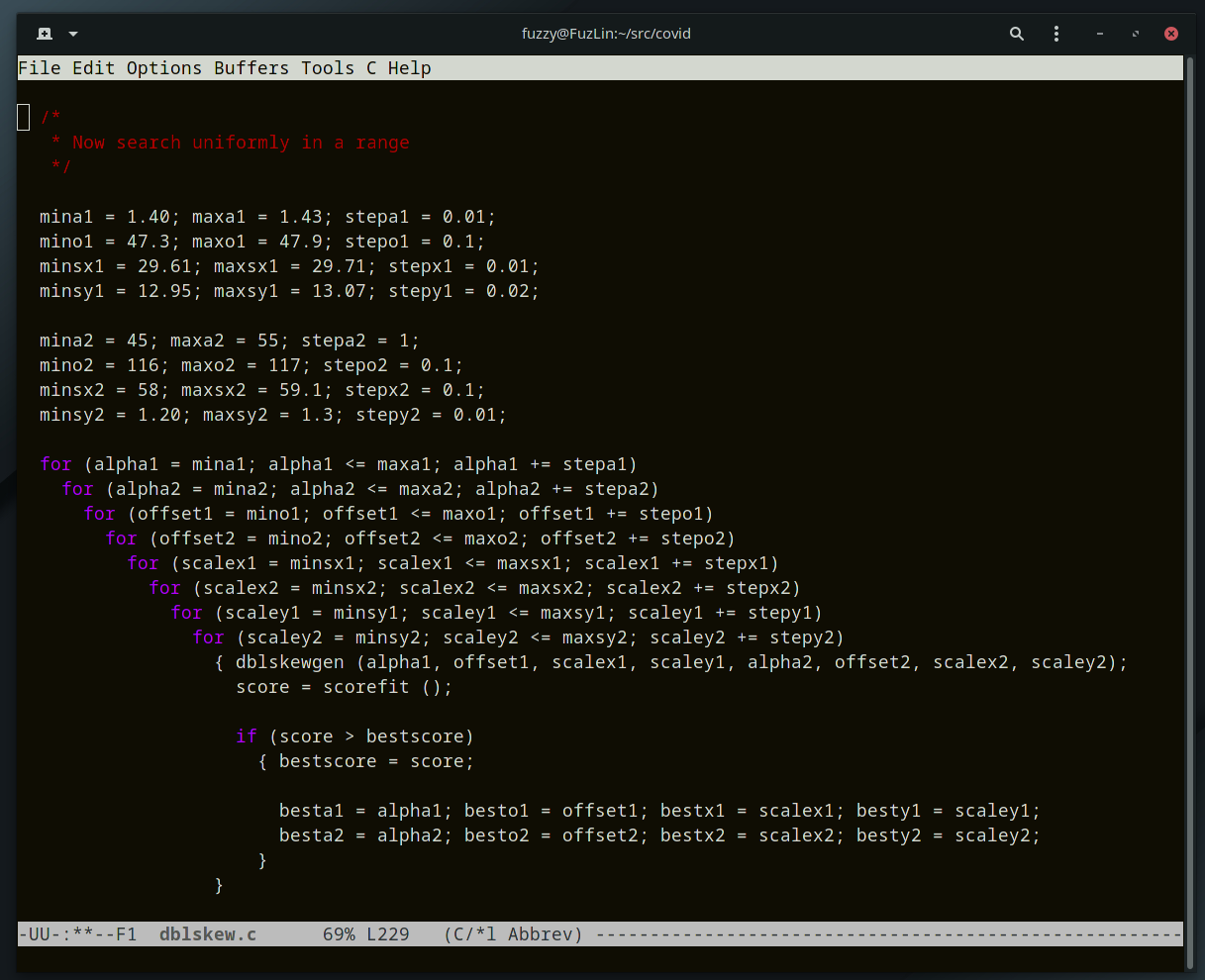The single-wave search takes a few seconds to a minute on a modest laptop, but it takes several runs to get a solution, because you have to start searching a large area (a hyper-rectangle), and then gradually reduce the range for each of the four dimensions.

The double-wave search takes a lot longer, taking 10-20 minutes on that same modest laptop. And again it took a few runs, narrowing down the ranges for each parameter to get a good fit.

 Daily new cases, United StatesAugust 28, 2020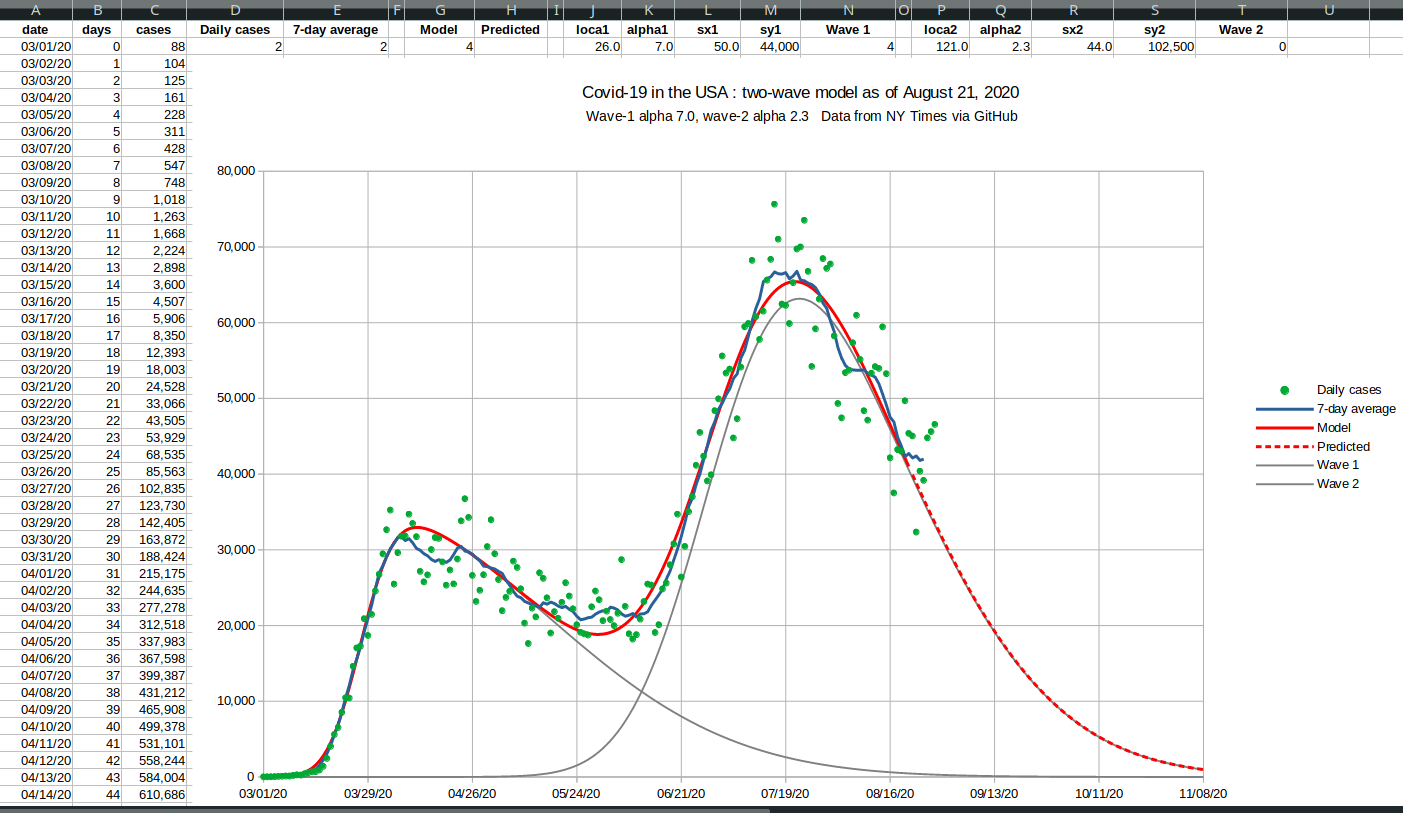Daily deaths, United StatesAugust 28, 2020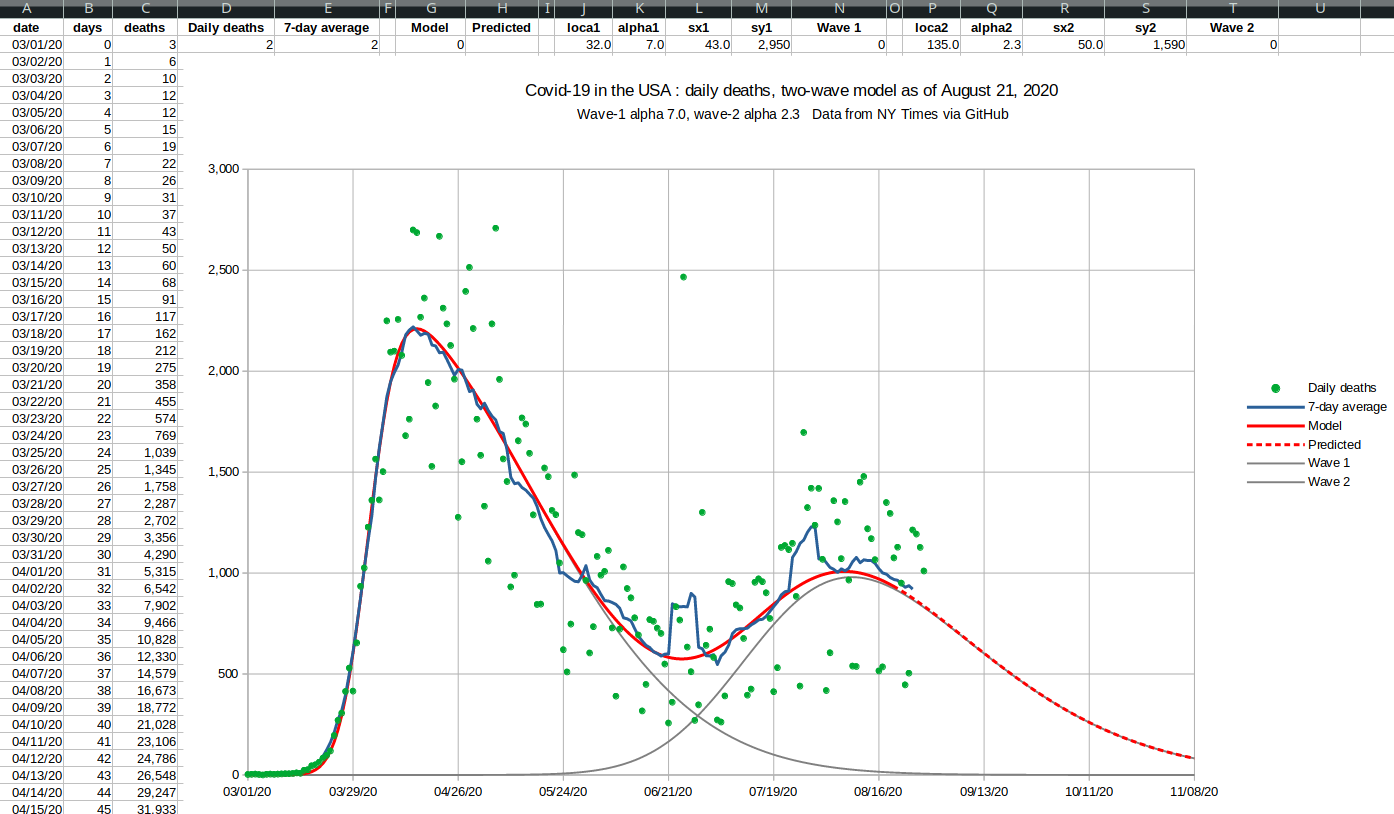During the fall I was spending an hour or so on each curve fit, and the eight nested for loops would examine between 50 million and 100 million curves. It was a lot of work, and the choice of where and how much to reduce the eight-dimensional rectangle was part of the problem.

### Higher Dimensions

 Starting in the fall, the cases started trending above the double-skew model, and the need for a three-wave curve fitter became apparent. But a brute force or uniform search was out of the question for twelve dimensional space. Even hill climbing is a challenge in that many dimensions, because there are 531,440 directions in which to move (allowing all the diagonals).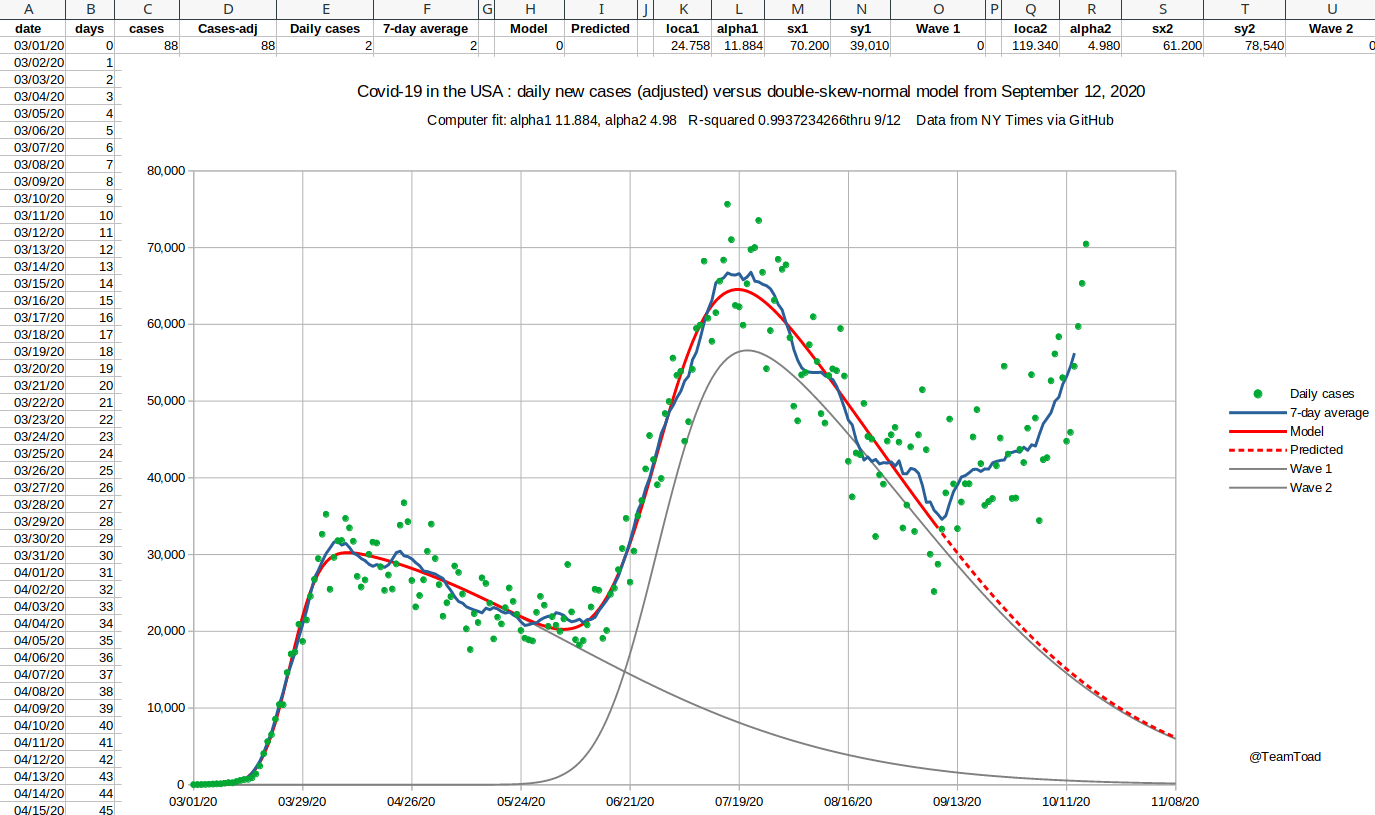### Genetic Algorithms

One effective method for optimizing in higher dimensions is a genetic algorithm, which I'd previously explored in my master's thesis, Maintaining Diversity in Genetic Search (AAAI-84).

To take advantage of the eight cores in my laptop computer, I modified the standard genetic algorithm to work in parallel. The gene pool was represented by a single array, divided into eight subpools, allowing each subpool to be managed by a single thread. In a multi-threaded architecture each thread shares memory with the other threads.

Each thread must be careful not to change memory being used by other threads. Since each subpool was manipulated by only one thread, each CPU core could work without affecting the work done by the other cores.

 I broke the usual evolutionary loop into 20 rounds. In each round a thread would be started for each subpool, and would run for a few thousand generations. At the end of the round all the threads would end, and the entire pool would be randomly shuffled. That way the best solutions would percolate throughout the whole gene pool, and be used by all the cores in the next round. You can see in the system monitor display on the right that for a time each CPU core was working at 100%, with brief periods where the only one core was working to shuffle the main pool. Three wave genetic search on eight cores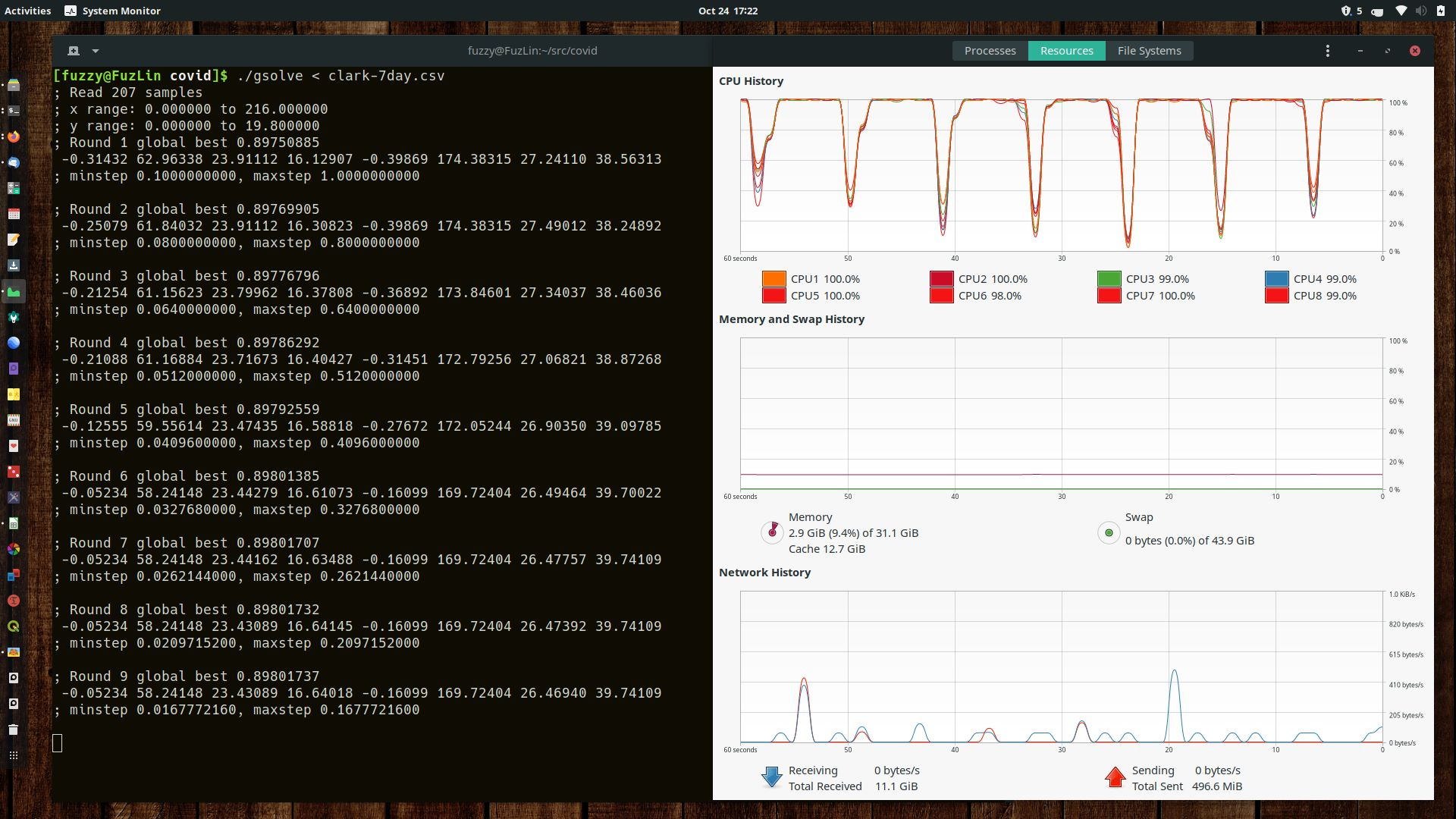### Details about the genetic curve fitter

Input

The input to the curve fitter is a list of X and Y floating point numbers representing the curve to be fitted.

Representation

Each possible solution curve is represented as a sum of N skew-normal curves, and in turn these are specified by 4×N double length floating point numbers. I am calling this sum the multiskew model.

Fitness

A possible solution is scored by evaluating the multiskew value for each X in the input, and using the sum of squares of the difference in the Y values from the input curve. That makes this a minimization problem, because the best solution has the least difference in the sum of squares from the curve being fitted.

Initialization

During the first round, each thread initializes its own subpool with randomly generated floating point numbers. For each gene a few thousand random curves are scored, and the best curve is saved.

One feature of genetic algorithms is that you can use knowledge about the domain to choose good initial values to improve the search. For example, when fitting a three-wave curve, you already know the basic shape of the first two waves, so you can set the first eight values close to the expected solution.

Evolution Model

After each subpool is populated, a generation consists of sorting the gene pool by fitness and then generating the next generation by choosing parents from the top 25% of genes. The top 25% are kept for the next generation, and the bottom 75% are replaced by new genes.

Reproductive Operators

To replace one of the bottom 75% genes, one or two parents are chosen and used to generate the next child. Each allele in a gene is just a floating point number, and every gene is just a vector in 4×N dimensions.
• cross-over: each allele is chosen from one of the two parents at random, making sure that atleast one value is chosen from each parent.

• averaging: this is a special operator that only makes sense for numberic alleles. Each value is just the average of the two parents. Instrumenting the program shows that the average operator actually generate new genes better than each parent more often than any other operator.

• mutation: applies to a single parent. I actually used two forms of mutation, big and small. A big mutation allowed a random change up to 2% up or down from the original value. A small mutation allowed a small amount based on the current grid size to be added ot subtracted from the parent value.

• hill-climbing: is another operator that makes sense when alleles are numeric. Given two parents, one has a lower score than the other. A vector from the lower to the higher parent is calculated, and then a loop generates successive children by adding that vector to the higher-scoring parent. This process continues until the score decreases, and the previous value is used as the next child. Instrumentation shows that hill-climbing doesn't generate better scores as often as the other operatos, but that when it works it can go tens or hundreds of steps in the right direction, and contributes to a much better solution.

Grid Search

For each major round, a rounding value is used to force each value to a grid. At first this grid is relatively coarse, with each allele rounded to just one digit after the decimal point. But as the rounds increase the grid is made finer and finer. The last round typically has a rounding value of 1000, which corresponds to three significant figures after the deciaml point.

Diversity

A common problem in genetic algorithms is convergence, where all the members of the gene pool are too similar, and new solutions are not generated by crossing them for the next generation. Mutation is one way to reintroduce diversity, but in my master's thesis I explored just forcing every new gene to be different from every other gene.

Of course, in a parallel-computing model, I could only enforce diversity within the subpool, since one thread cannot count on memory in another thread being useful.

This diversity combines with the grid search to enforce a broad spread of possible solutions early in the rounds, and as the grid size is reduced the gene pool is allowed to cluster closer and closer around the best solutions.

In this implementation I just added a diversity check, and if a new gene was too similar to one already in the subpool, it was discarded and a new child was generated. Sanity

As each new gene is generated, it is checked for sanity, because some values just don't make sense. For example, you can't have zero or negative values for either the X-scale or Y-scale, and the Y-scale can't be larger than the population of the country. If a gene fails the sanity check it is discarded, and that saves the expensive computation of scoring the gene (each score requires evaluating the multiskew value for hundreds of X coordinates, involving lots of floating point math).

For this epidemic model, sanity also involved making sure that none of the individual waves started before the first day of the input numbers.

### The Fourth Dimension

In the late fall of 2020 I started using four waves to model the case numbers in the United States, because there was a small but definite "bump" over September that I call "Wave Two and a Half".

Fortunately once you've modified your code to use a loop to represent each wave, it's just a change of N from 3 to 4.

 Daily new cases, United StatesDecember 24, 2020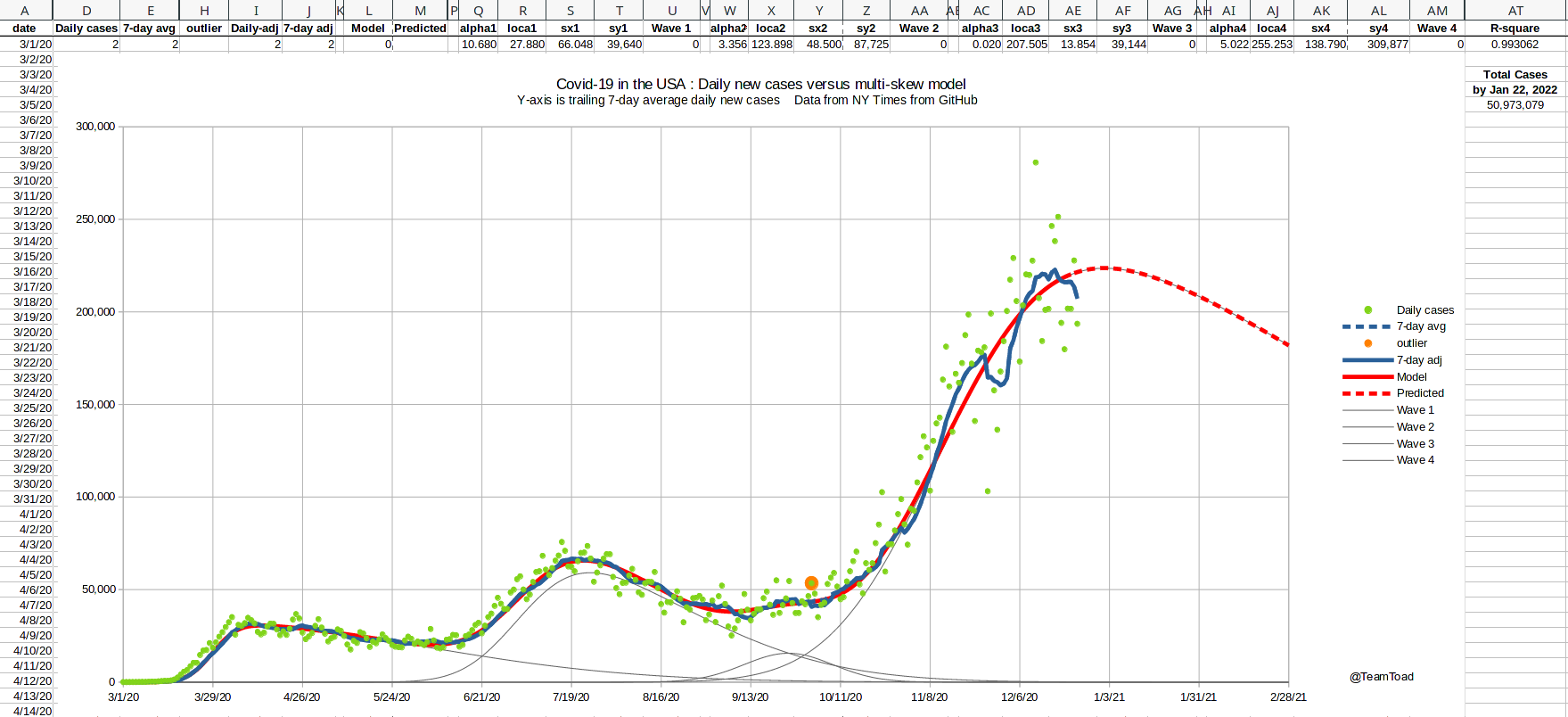Daily deaths, United StatesDecember 24, 2020### The Peak-Skew Transform

One of the reasons I needed to automate the curve fitter was that the four key parameters are not really independent. Changing the value of alpha moves the peak of the curve up or down and left or right. Changing the horizontal scale also moves the loca value left or right.

To reduce these dependencies and make searching the space easier, I added a transformation to use peak X and peak Y in place of loca and scale-Y during the genetic search.

There is no closed form equation I know off to do this transform, so it involved coding a trisection search to convert one to the other, but the scoring function is so expensive that the transform doesn't really slow the program down. But the genetic algorithm converges much faster to a solution since a mutation is likely to be "better" without the dependencies.

### Upper-bound modeling

 Three major holidays occured in the growth phase of the third wave: Thanksgiving, Christmas, and New Year's. Because of the likelihood of underreporting cases during these holidays, and the possibility of delayed reporting of deaths, I needed to adjust the curve fitter to handle missing data (you can see the notches in the graph shown at the right showing missing cases). I used an upper-bound least-squares scoring function for the mid-January curve fitting run. Basically the actual squared error was used if the model under-predicted the data point, but only 25% of the distance was used if the model over-predicted the data point. This modification was only used for data after November 16th, to allow for missing data from Thanksgiving on. This change in scoring causes the fitted curve to skim the top of the data points, and to ignore the valleys. That result is shown by the red line in the graph shown to the right. The R-squared measures are still reported using standard least-squares.The 2020 holiday seasonDaily new cases

## Summary

The epicurves for both daily new cases and daily deaths from the current Coronavirus epidemic can be closely modeled as a sum of skew-normal curves each presenting one wave of the epidemic. The parameters for these curves can be estimated effectively using a genetic algorithm as described.